# Themes

### Plot Themes

Both ggsurvfit() and ggcuminc() use theme_ggsurvfit_default() as the default {ggplot2} theme, which is similar to ggplot2::theme_bw(). While this theme provides beautiful figures, you may want to modify it.

You can use the ggsurvfit(theme=) argument to change the default theme. You can pass any {ggplot2} theme to this argument, or simply add a theme using typical {ggplot2} syntax.

library(ggsurvfit)
library(patchwork)

gg_theme_default1 <-
survfit2(Surv(time, status) ~ surg, data = df_colon) %>%
ggsurvfit(theme = theme_ggsurvfit_default()) +
labs(title = "Using theme= argument")

gg_theme_default2 <-
survfit2(Surv(time, status) ~ surg, data = df_colon) %>%
ggsurvfit(theme = NULL) +
theme_ggsurvfit_default() +
labs(title = "Using ggplot +")

gg_theme_default1 + gg_theme_default2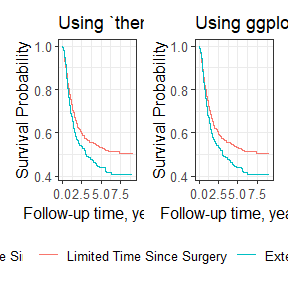As you can see, either method of passing the theme results in an identical figure. You can easily apply any user-created theme or any theme that exported with the ggplot2 package. To construct a custom theme, include ggplot2 calls in a list as in the example below.

survfit2(Surv(time, status) ~ surg, data = df_colon) %>%
ggsurvfit(theme = list(theme_classic(), theme(legend.position = "top"))) +
labs(title = "Custom Plot Theme")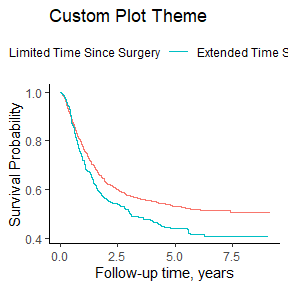### Risk Table Themes

Similar to the plots above, the risk tables also come with a default theme. To understand how to use risk table themes, one must first understand what a risk table is. Each risk table that is placed below a plot is itself a ggplot created with ggplot2::geom_text().

Unlike the plot themes, the risk table themes can only be passed via add_risktable(theme=).

survfit2(Surv(time, status) ~ surg, data = df_colon) %>%
ggsurvfit() +
risktable_stats = "n.risk",
theme = theme_risktable_default()
)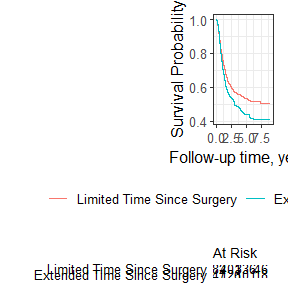A typical theme appropriate for a risk table plot will remove grid lines and provide a clean area to display the numbers at risk. In the risk table above, the strata levels are y-axis labels, and “At Risk” is the plot title.

Another risk table theme adds a box around the statistics presented.

survfit2(Surv(time, status) ~ surg, data = df_colon) %>%
ggsurvfit() +
risktable_stats = "n.risk",
theme = theme_risktable_boxed()
)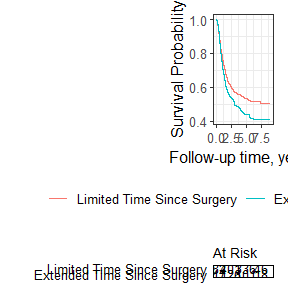It’s possible to replace the stratum levels with a symbol, which is particularly helpful when you have long group labels.

survfit2(Surv(time, status) ~ surg, data = df_colon) %>%
ggsurvfit() +
add_risktable_strata_symbol()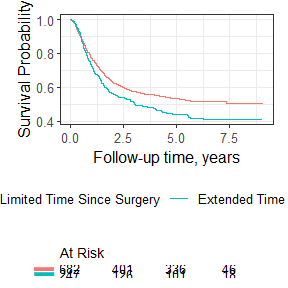When customizing the risk table themes and using add_risktable_strata_symbol(), note that the symbol is added with ggplot2::scale_y_discrete(); including a theme that uses the discrete y scale could cause a conflict.